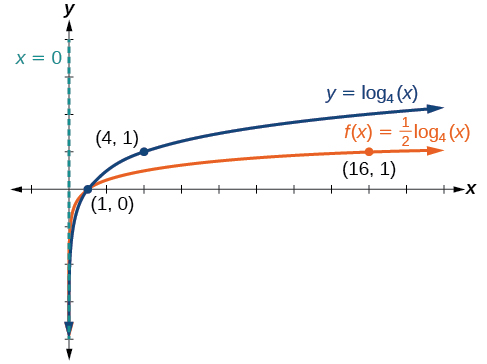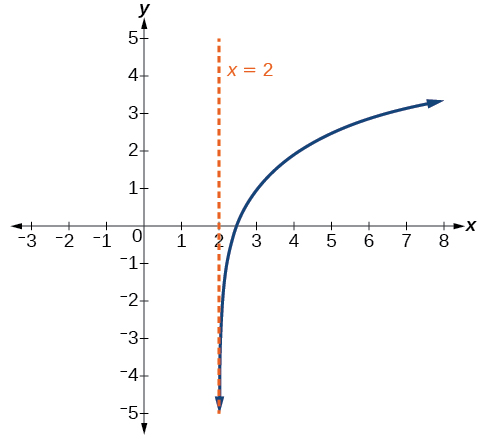# 6.4 Graphs of logarithmic functions  (Page 5/8)

 Page 5 / 8

Given a logarithmic function with the form $\text{\hspace{0.17em}}f\left(x\right)=a{\mathrm{log}}_{b}\left(x\right),$ $a>0,$ graph the translation.

1. Identify the vertical stretch or compressions:
• If $\text{\hspace{0.17em}}|a|>1,$ the graph of $\text{\hspace{0.17em}}f\left(x\right)={\mathrm{log}}_{b}\left(x\right)\text{\hspace{0.17em}}$ is stretched by a factor of $\text{\hspace{0.17em}}a\text{\hspace{0.17em}}$ units.
• If $\text{\hspace{0.17em}}|a|<1,$ the graph of $\text{\hspace{0.17em}}f\left(x\right)={\mathrm{log}}_{b}\left(x\right)\text{\hspace{0.17em}}$ is compressed by a factor of $\text{\hspace{0.17em}}a\text{\hspace{0.17em}}$ units.
2. Draw the vertical asymptote $\text{\hspace{0.17em}}x=0.$
3. Identify three key points from the parent function. Find new coordinates for the shifted functions by multiplying the $\text{\hspace{0.17em}}y\text{\hspace{0.17em}}$ coordinates by $\text{\hspace{0.17em}}a.$
4. Label the three points.
5. The domain is $\text{\hspace{0.17em}}\left(0,\infty \right),$ the range is $\text{\hspace{0.17em}}\left(-\infty ,\infty \right),$ and the vertical asymptote is $\text{\hspace{0.17em}}x=0.$

## Graphing a stretch or compression of the parent function y = log b ( x )

Sketch a graph of $\text{\hspace{0.17em}}f\left(x\right)=2{\mathrm{log}}_{4}\left(x\right)\text{\hspace{0.17em}}$ alongside its parent function. Include the key points and asymptote on the graph. State the domain, range, and asymptote.

Since the function is $\text{\hspace{0.17em}}f\left(x\right)=2{\mathrm{log}}_{4}\left(x\right),$ we will notice $\text{\hspace{0.17em}}a=2.$

This means we will stretch the function $\text{\hspace{0.17em}}f\left(x\right)={\mathrm{log}}_{4}\left(x\right)\text{\hspace{0.17em}}$ by a factor of 2.

The vertical asymptote is $\text{\hspace{0.17em}}x=0.$

Consider the three key points from the parent function, $\text{\hspace{0.17em}}\left(\frac{1}{4},-1\right),$ $\left(1,0\right),\text{\hspace{0.17em}}$ and $\text{\hspace{0.17em}}\left(4,1\right).$

The new coordinates are found by multiplying the $\text{\hspace{0.17em}}y\text{\hspace{0.17em}}$ coordinates by 2.

Label the points $\text{\hspace{0.17em}}\left(\frac{1}{4},-2\right),$ $\left(1,0\right)\text{\hspace{0.17em}},$ and $\text{\hspace{0.17em}}\left(4,\text{2}\right).$

The domain is $\text{\hspace{0.17em}}\left(0,\text{\hspace{0.17em}}\infty \right),$ the range is $\text{\hspace{0.17em}}\left(-\infty ,\infty \right),\text{\hspace{0.17em}}$ and the vertical asymptote is $\text{\hspace{0.17em}}x=0.\text{\hspace{0.17em}}$ See [link] .

The domain is $\text{\hspace{0.17em}}\left(0,\infty \right),$ the range is $\text{\hspace{0.17em}}\left(-\infty ,\infty \right),$ and the vertical asymptote is $\text{\hspace{0.17em}}x=0.$

Sketch a graph of $\text{\hspace{0.17em}}f\left(x\right)=\frac{1}{2}\text{\hspace{0.17em}}{\mathrm{log}}_{4}\left(x\right)\text{\hspace{0.17em}}$ alongside its parent function. Include the key points and asymptote on the graph. State the domain, range, and asymptote.The domain is $\text{\hspace{0.17em}}\left(0,\infty \right),$ the range is $\text{\hspace{0.17em}}\left(-\infty ,\infty \right),$ and the vertical asymptote is $\text{\hspace{0.17em}}x=0.$

## Combining a shift and a stretch

Sketch a graph of $\text{\hspace{0.17em}}f\left(x\right)=5\mathrm{log}\left(x+2\right).\text{\hspace{0.17em}}$ State the domain, range, and asymptote.

Remember: what happens inside parentheses happens first. First, we move the graph left 2 units, then stretch the function vertically by a factor of 5, as in [link] . The vertical asymptote will be shifted to $\text{\hspace{0.17em}}x=-2.\text{\hspace{0.17em}}$ The x -intercept will be $\text{\hspace{0.17em}}\left(-1,0\right).\text{\hspace{0.17em}}$ The domain will be $\text{\hspace{0.17em}}\left(-2,\infty \right).\text{\hspace{0.17em}}$ Two points will help give the shape of the graph: $\text{\hspace{0.17em}}\left(-1,0\right)\text{\hspace{0.17em}}$ and $\text{\hspace{0.17em}}\left(8,5\right).\text{\hspace{0.17em}}$ We chose $\text{\hspace{0.17em}}x=8\text{\hspace{0.17em}}$ as the x -coordinate of one point to graph because when $\text{\hspace{0.17em}}x=8,\text{\hspace{0.17em}}$ $\text{\hspace{0.17em}}x+2=10,\text{\hspace{0.17em}}$ the base of the common logarithm.

The domain is $\text{\hspace{0.17em}}\left(-2,\infty \right),$ the range is $\text{\hspace{0.17em}}\left(-\infty ,\infty \right),$ and the vertical asymptote is $\text{\hspace{0.17em}}x=-2.$

Sketch a graph of the function $\text{\hspace{0.17em}}f\left(x\right)=3\mathrm{log}\left(x-2\right)+1.\text{\hspace{0.17em}}$ State the domain, range, and asymptote.The domain is $\text{\hspace{0.17em}}\left(2,\infty \right),$ the range is $\text{\hspace{0.17em}}\left(-\infty ,\infty \right),$ and the vertical asymptote is $\text{\hspace{0.17em}}x=2.$

## Graphing reflections of f ( x ) = log b ( x )

When the parent function $\text{\hspace{0.17em}}f\left(x\right)={\mathrm{log}}_{b}\left(x\right)\text{\hspace{0.17em}}$ is multiplied by $\text{\hspace{0.17em}}-1,$ the result is a reflection about the x -axis. When the input is multiplied by $\text{\hspace{0.17em}}-1,$ the result is a reflection about the y -axis. To visualize reflections, we restrict $\text{\hspace{0.17em}}b>1,\text{\hspace{0.17em}}$ and observe the general graph of the parent function $\text{\hspace{0.17em}}f\left(x\right)={\mathrm{log}}_{b}\left(x\right)\text{\hspace{0.17em}}$ alongside the reflection about the x -axis, $\text{\hspace{0.17em}}g\left(x\right)={\mathrm{-log}}_{b}\left(x\right)\text{\hspace{0.17em}}$ and the reflection about the y -axis, $\text{\hspace{0.17em}}h\left(x\right)={\mathrm{log}}_{b}\left(-x\right).$

## Reflections of the parent function y = log b ( x )

The function $\text{\hspace{0.17em}}f\left(x\right)={\mathrm{-log}}_{b}\left(x\right)$

• reflects the parent function $\text{\hspace{0.17em}}y={\mathrm{log}}_{b}\left(x\right)\text{\hspace{0.17em}}$ about the x -axis.
• has domain, $\text{\hspace{0.17em}}\left(0,\infty \right),$ range, $\text{\hspace{0.17em}}\left(-\infty ,\infty \right),$ and vertical asymptote, $\text{\hspace{0.17em}}x=0,$ which are unchanged from the parent function.

The function $\text{\hspace{0.17em}}f\left(x\right)={\mathrm{log}}_{b}\left(-x\right)$

• reflects the parent function $\text{\hspace{0.17em}}y={\mathrm{log}}_{b}\left(x\right)\text{\hspace{0.17em}}$ about the y -axis.
• has domain $\text{\hspace{0.17em}}\left(-\infty ,0\right).$
• has range, $\text{\hspace{0.17em}}\left(-\infty ,\infty \right),$ and vertical asymptote, $\text{\hspace{0.17em}}x=0,$ which are unchanged from the parent function.

what are odd numbers
numbers that leave a remainder when divided by 2
Thorben
1,3,5,7,... 99,...867
Thorben
7%2=1, 679%2=1, 866245%2=1
Thorben
the third and the seventh terms of a G.P are 81 and 16, find the first and fifth terms.
if a=3, b =4 and c=5 find the six trigonometric value sin
pls how do I factorize x⁴+x³-7x²-x+6=0
in a function the input value is called
how do I test for values on the number line
if a=4 b=4 then a+b=
a+b+2ab
Kin
commulative principle
a+b= 4+4=8
Mimi
If a=4 and b=4 then we add the value of a and b i.e a+b=4+4=8.
Tariq
what are examples of natural number
an equation for the line that goes through the point (-1,12) and has a slope of 2,3
3y=-9x+25
Ishaq
show that the set of natural numberdoes not from agroup with addition or multiplication butit forms aseni group with respect toaaddition as well as multiplication
x^20+x^15+x^10+x^5/x^2+1
evaluate each algebraic expression. 2x+×_2 if ×=5
if the ratio of the root of ax+bx+c =0, show that (m+1)^2 ac =b^2m
By the definition, is such that 0!=1.why?
(1+cosA+IsinA)(1+cosB+isinB)/(cos@+isin@)(cos$+isin$)
hatdog
Mark
jaks
Ryan

#### Get Jobilize Job Search Mobile App in your pocket Now!ByByBy Stephen VoronBy OpenStaxBy Stephen VoronBy Mucho MizindukoBy OpenStaxBy Madison ChristianBy Brenna FikeBy Stephen VoronBy Ellie BanfieldBy OpenStax